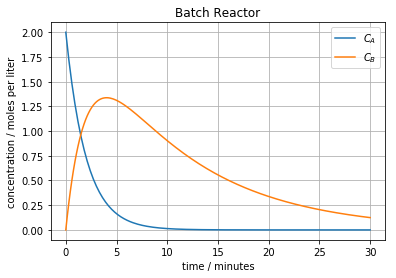This notebook contains material from ND-Pyomo-Cookbook; content is available on Github. The text is released under the CC-BY-NC-ND-4.0 license, and code is released under the MIT license.

# 6.2 Maximizing Concentration of an Intermediate in a Batch Reactor¶

Keywords: ipopt usage, scipy.minimize_scalar, scipy.odeint, unconstrained optimization

A desired product $B$ is produced as intermediate in a series reaction

\begin{align} A \overset{k_A}{\longrightarrow} B \overset{k_B}{\longrightarrow} C \end{align}

where $A$ is a raw material and $C$ is a undesired b y-product. The reaction operates at temperature where the rate constants are $k_A = 0.5\ \mbox{min}^{-1}$ and $k_A = 0.1\ \mbox{min}^{-1}$. The raw material is available as a solution with concenration $C_{A,f} = 2.0\ \mbox{moles/liter}$.

A 100 liter tank is available which can be operated as a batch reactor. At what point should the operation be terminated in order to maximize the concentration of $B$?

## 6.2.1 Imports¶

In :
%matplotlib inline

import matplotlib.pyplot as plt
import numpy as np
from scipy.integrate import odeint
from scipy.optimize import minimize_scalar

import shutil
import sys
import os.path

if not shutil.which("pyomo"):
!pip install -q pyomo
assert(shutil.which("pyomo"))

if not (shutil.which("ipopt") or os.path.isfile("ipopt")):
!wget -N -q "https://ampl.com/dl/open/ipopt/ipopt-linux64.zip"
!unzip -o -q ipopt-linux64
else:
try:
!conda install -c conda-forge ipopt
except:
pass

assert(shutil.which("ipopt") or os.path.isfile("ipopt"))
from pyomo.environ import *
from pyomo.dae import *


## 6.2.2 Mathematical model¶

A material balance for an isothermal stirred batch reactor with a volume $V = 40$ liters and an initial concentration $C_{A,f}$ is given by

\begin{align} V\frac{dC_A}{dt} & = - V k_A C_A \\ V\frac{dC_B}{dt} & = V k_A C_A - V k_B C_B \end{align}

Eliminating the common factor $V$

\begin{align} \frac{dC_A}{dt} & = - k_A C_A \\ \frac{dC_B}{dt} & = k_A C_A - k_B C_B \end{align}

With an initial concentration $C_{A,f}$. A numerical solution to these equations is shown in the following cell.

In :
V = 40     # liters
kA = 0.5   # 1/min
kB = 0.1   # l/min
CAf = 2.0  # moles/liter

def batch(X, t):
CA, CB = X
dCA_dt = -kA*CA
dCB_dt = kA*CA - kB*CB
return [dCA_dt, dCB_dt]

t = np.linspace(0,30,200)
soln = odeint(batch, [CAf,0], t)
plt.plot(t, soln)
plt.xlabel('time / minutes')
plt.ylabel('concentration / moles per liter')
plt.title('Batch Reactor')
plt.legend(['$C_A$','$C_B$'])
plt.grid(True)## 6.2.3 Optimization with scipy.minimize_scalar¶

To find the maximum value, we first write a function to compute $C_B$ for any value of time $t$.

In :
def CB(tf):
soln = odeint(batch, [CAf, 0], [0, tf])
return soln[-1]


We gain use minimize_scalar to find the value of $t$ that minimizes the negative value of $C_B(t)$.|

In :
minimize_scalar(lambda t: -CB(t), bracket=[0,50])

Out:
     fun: -1.3374806339222158
nfev: 23
nit: 19
success: True
x: 4.023594924340666
In :
tmax = minimize_scalar(lambda t: -CB(t), bracket=[0,50]).x

print('Concentration c_B has maximum', CB(tmax), 'moles/liter at time', tmax, 'minutes.')

Concentration c_B has maximum 1.3374806339222158 moles/liter at time 4.023594924340666 minutes.


## 6.2.4 Solution using Pyomo¶

The variable to be found is the time $t_f$ corresponding to the maximum concentration of $B$. For this purpose we introduce a scaled time

$$\tau = \frac{t}{t_f}$$

so that $\tau=1$ as the desired solution. The problem then reads

\begin{align} \max_{t_f} C_B(\tau=1) \end{align}

subject to

\begin{align} \frac{dC_A}{d\tau} & = - t_f k_A C_A \\ \frac{dC_B}{d\tau} & = t_f(k_A C_A - k_B C_B) \end{align}

The solution to this problem is implemented as a solution to the following Pyomo model.

In :
V   = 40    # liters
kA  = 0.5   # 1/min
kB  = 0.1   # l/min
cAf = 2.0   # moles/liter

m = ConcreteModel()

m.tau = ContinuousSet(bounds=(0, 1))

m.tf = Var(domain=NonNegativeReals)
m.cA = Var(m.tau, domain=NonNegativeReals)
m.cB = Var(m.tau, domain=NonNegativeReals)

m.dcA = DerivativeVar(m.cA)
m.dcB = DerivativeVar(m.cB)

m.odeA = Constraint(m.tau,
rule=lambda m, tau: m.dcA[tau] == m.tf*(-kA*m.cA[tau]) if tau > 0 else Constraint.Skip)
m.odeB = Constraint(m.tau,
rule=lambda m, tau: m.dcB[tau] == m.tf*(kA*m.cA[tau] - kB*m.cB[tau]) if tau > 0 else Constraint.Skip)

m.ic = ConstraintList()

Concentration c_B has maximum 1.3374805810221078 moles/liter at time 4.023594178375688 minutes.